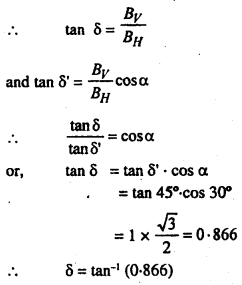# Bihar Board 12th Physics Numericals Important Questions with Solutions

BSEB Bihar Board 12th Physics Numerical Important Questions Numericals are the best resource for students which helps in revision.

## Bihar Board 12th Physics Numericals Important Questions with Solutions

Question 1.
A Convex lens of focal length 20 cm. in air is dipped in water. What will be its focal length in water? The refractive indices of glass and water are $$\frac{3}{2}$$ and $$\frac{4}{3}$$ respectively.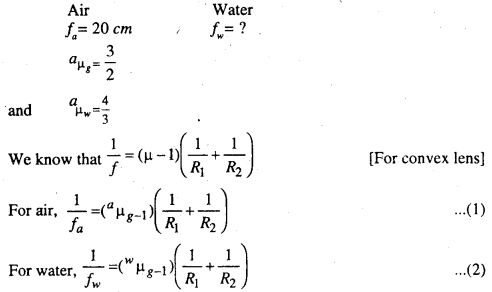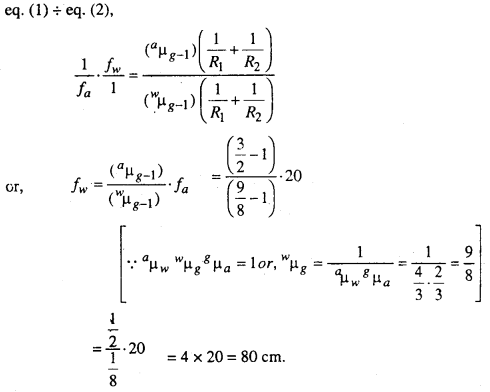Question 2.
Inside a glass sphere of radius 3 cm, there is a small air bubble. The bubble appears 2 cm. below the outer surface. What is the real distance of the bubble from the surface. Refractive index glass is 1.5.
Let the centre of the sphere be at O. A and A’ are real and apparent position of the bubble.
From question
r = -3 cm.
V = BA’ = -2cm
Since the refraction takes place from glass to air
∴ µ = 1.5 and µ2 = 1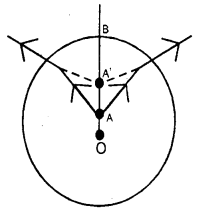Question 3.
A man cannot see objects lying within 1 metre. Calculate the power of that lens by which he can read a book at a distance of 0.5 m.
From question, u = -0.5 m
V = -1m
f = ?
From lens formula,
$$\frac{1}{v}-\frac{1}{u}=\frac{1}{f}$$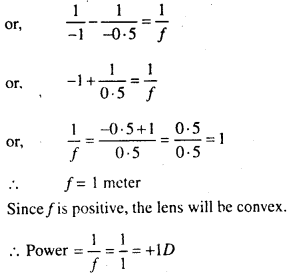Question 4.
A Compound microscope has an objective and an eye piece of focal length 4 cm and 10 cm. respectively. When an object is kept at a distance of 5 cm. from the objective then final image is formed at the least distance of distinct vision. Calculate the magnification and length of the microscope.
For objective, f0 = 4 cm and u0 = -5 cm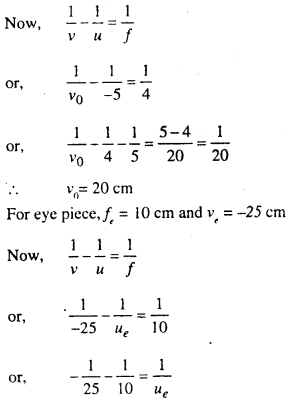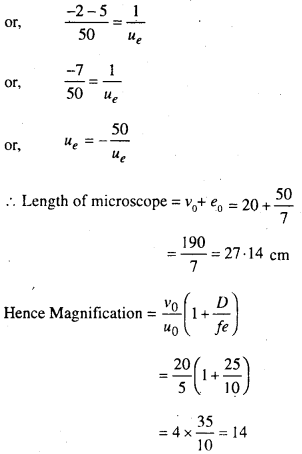Question 5.
A Crown glass prism of angle 10° (µv= 154 and µR = 151) is combined with a flint glass prism (µv= 1·66 and µR = 1·62) so as to produce deviation without dispersion of without dispersion of white light. Calculate the angle of flint prism. If refractive index of two prisms for yellow colours are 1·53 and 1·63 respectively then calculate total deviation produced.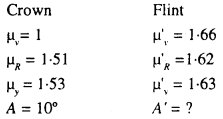Total deviation = ?
Dispersion from crown prism = (µv – µR) A
= (1·54 – 1·57). 10
= 0·03 × 10 = 0·3 ……….(1)
Dispersion from flint prism = (1·66 – 1·62) A’
= 0·04A’ …………..(2)
Due to production of deviation without dispersion,
Eq. (1) = -Eq. (2),
0·3 = -0·04A’
or, A’ = $$-\frac{0 \cdot 3}{0 \cdot 04}$$ = -7·5°
or, A’ = $$-\frac{(0 \cdot 3)}{0 \cdot 4}$$ = -7·5°
∴ Total deviation = deviation from crown + deviation from flint
= A(µy – 1) + A'(M’y – 1)
= (1·53 – 1) + (-7·5) (1·63 – 1)
= 10 × 0·53 – 7·5 × 0·63
= 0·575°

Question 6.
In an interference experiment the distance between the two slits is 0·05 cm. If the distance of the score from the slit is increased by 40 cm, the fringe width is found to increases by 0·04 cm. What is the wavelength of light.
In first case, d = 0·05 cm
∴ λ = $$\frac{0 \cdot 05 \beta}{D}$$ …………(1)
Now, when the distance of the screen from the slit (D) is increased by 40 cm, then β changes to (β + 0·04) cm
∴ λ = $$\frac{(\beta+0 \cdot 04) \times 0 \cdot 05}{D+40}$$ ……….(2)
From equation (1) and (2), we have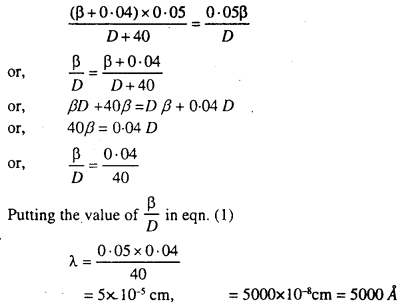Question 7.
Calculate the equivalent capacity in the given fig. across M and N.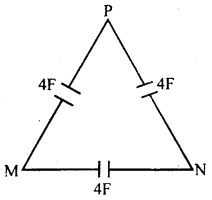Equivalent capacity in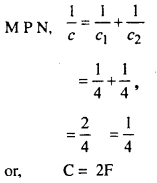This C is connected with 4F in parallel.
∴ Equivalent capacity across M and N = 2 + 4 = 6F

Question 8.
Calculate the equivalence capacity in the given fig. across M and N (b) M and B.
(a) Equivalent capacity in M A N,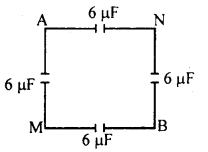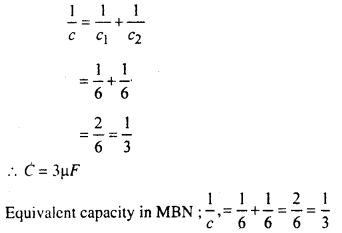∴ c’ = 3µF
∴ Equivalent capacity across M and N
= 3 + 3 = 6µF

(b) Equivalent capacity in MANB,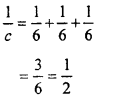or, C = 2µF
Again C and 6F are in parallel.
Hence Equivalent capacity across M and B. = 2 + 6 = 8µF

Question 9.
Calculate the equivalent capacity in the given fig. across the points (a) M and B (b) M and N.
(a) Equivalent capacity in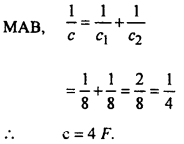Again Equivalent capacity in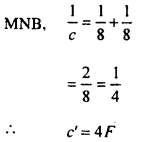Similarly Equivalent capacity in MB = 4F
All those are in parallel
∴ Total Equivalent capacity across M and B
= 4F + 4F + 4F = 12F

(b) Equivalent capacity of MAB in series = 4F
Again Equivalent capacity of MB in series = 4F
These two are in parallel, hence Equivalent capacity = 4 + 4 = 8F
which in series with BN (8F)
∴ Equivalent capacity = 4F
Which in parallel with MN (8F)
∴ Equivalent capacity across M and N = 4 + 8
= 12 F.

Question 10.
In the given figures calculate the equivalent capacity between A and C.
The given figure is on the principle of wheat stone bridge in which the potential of B and D are same. Hence 4F is eneffactive.Hence Equivalent capacity in ABC,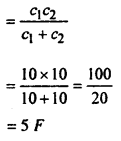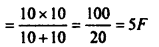But these two equivalent capacity are in parallel
∴ Equivalent capacity between A and C = 5 + 5 = 10 F

Question 11.
In the figure shown calculated the potential diff. (p.d.) across the capacitance c2. The capacity of c1,c2 and c3 are 20F, 30F and 15F and the potential of point A is 90 V.
Since c1, c2 and c3 are in series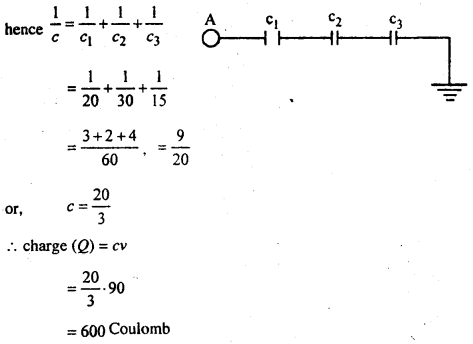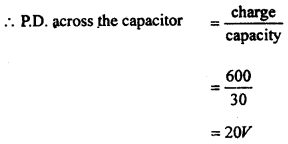Question 12.
In Young’s experiment, it is found that width of 20 fringes if 19·6 mm. If the fringes are observed at 1·25 m from the slit which are 0·75 mm apart, find the wavelength of light used.Question 13.
A charge of 5 × 10-8 coulomb is brought from a point M to point N. 8 × 10-4. Joule work has to be performed in it. If the potential M be -2000 volt, then calculate the potential at N.
From question, charge = 5 × 10-8 C
work = 8 × 10-4J
Potential at M = -2000 volt
Potential at N = ?

Let us suppose that the potential at N = V
∴ Potential difference betwen M and N = V – (-2000)
= V + 2000
Now, work done = charge × potential difference
8 × 10-4 = 5 × 10-8 (ν + 2000)
or, ν + 2000 = $$\frac{8 \times 10^{-4}}{5 \times 10^{-8}}$$ = $$\frac{80000}{5}$$
or, ν = 16000 – 2000 = 14000 volt

Question 14.
27 drops of mercury of same size make one big drop. Find out the potential of big drop. One small drop has potential 3 volt.
Let charge on a smaller drop = q
radius on a smaller drop = r
From question, potential at one small drop = 3 volt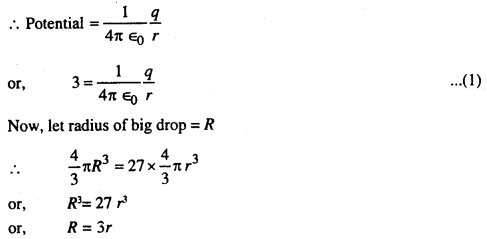Question 15.
Calculate the intensity of the field at a point distant 10 cm. From a point charge. The potential at that point is 3000 volt.
Here ν = 3000 volt
r = 10 cm = 0·1 mQuestion 16.
Three capacitors of capacitance 4, 3 and 2 units respectively are connected in such a way that the first and second are connected series and third in parallel with them. Calculate the resultant capacitance.
C1 = 4 unit
C2 = 3 unit
C3 = 2 unit
As the two capacitors are connected in series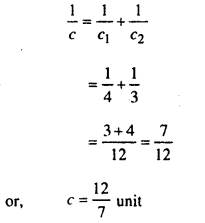The third capacitor of capacitance 2 unit is connected in parallel to it.
∴ Resultant capacitance = c + c3
= $$\frac{12}{7}$$ + 2
= $$\frac{26}{7}$$ = 3·71 unit.

Question 17.
The area of the plates of a parallel plate air capacitors is 10-2 square metre and the distance between the plate is 0·7 cm. If the potential difference between the plates is 66 volts, calculate the charge on the capacitor.
From question,
A = 10-2 m2
d = 0·7 cm = 0·7 x 10-2 m
ν = 66 volts
Charge (Q) = ?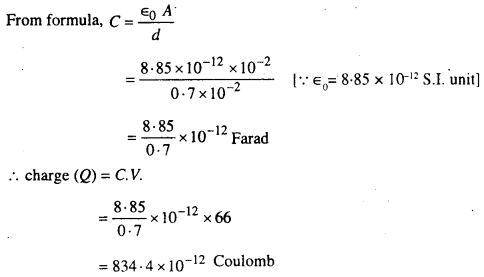Question 18.
The length of a potentiometer wire is 2 mitre and resistance is 4 ohm. Current flows in it from a cell of e.m.f. 2 volt and internal resistance 1 ohm. If a standard cell of e.m.f. 1·08 volt be joined where will be balance point obtained.
Lenght of the wire = 2 metre = 200 cm.
Resistance = 4 ohm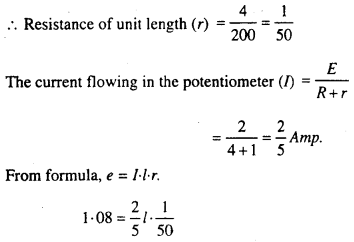or, l = $$\frac{1 \cdot 08 \times 5 \times 50}{2}$$ = 135 cm
∴ Length of balance point = 135 cm.

Question 19.
Two cells of e.m.f. 1·5 volt and 2 volt and internal resistance 1 ohm and 2 ohm are connected in parallel with an external 5 ohm resistance. Find the current in each branch of this network.
Applying Kirchoffs second law in closed circuit E1 ABE1Multiplying eq. (1) by 5 and eq. (2) by 6, we getPutting the value of I2 in eq. (2), we get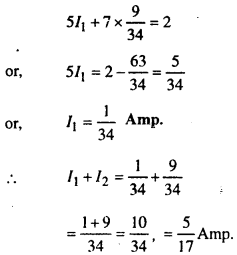Question 20.
When a potential difference of 200 volt is established between the ends of a conductor the current flowing in its is 4·2 amp. And heat produced per second in 840 Joule. Find the value of J.
From question.
V = 200 volt
I = 4·2 amp.
t = 1 second
H = 840 J
From Formula, w = Vlt
= 200 × 4·2 × 1 = 840 Joule
Now, w =J·H
840 = J·840
or, J = 1.

Question 21.
Two conductors of resistance in the ratio of 3 : 4 are joined in parallel. If the potential difference between the ends of the conductor be constant, compare the heat produce in the two conductors.
Let us suppose that the resistance of two conductors are R1 and R2. Its current are C1 and C2 respectively.From constant potential difference = C1R1 = C2R2
∴ C1R1 = C2R2
or, $$\frac{C_{1}}{C_{2}}=\frac{R_{1}}{R_{2}}=\frac{4}{3}$$
Heat produced in first conductors (H1) = C21R1t
Heat produced in second conductors (H2) = C22R2t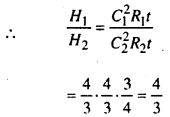Question 22.
A galvanometer baling 10 Ohm resistance given full scale deflection by a cur rent of 0·01 Amp. How this galvanometer may be made (i) an ammeter of 10 amp. Reading (ii) a voltmeter of 100 volt reading?
From question, G = 10 Ohm
Ig = 0.01 Amp
Total current (I) = 10 Amp.

(i) Let us suppose that the resistance of shunt used is S.Hence if the galv. Be shunted by a resistance of 0·01001 Ohm, it will become an ammeter.

(ii) Let a high resistance R is connected in series with galvanometer.
From Ohm’s law,
0·01 = $$\frac{100}{10+R}$$
or, 0·01 R = 100
or, 0·01 R = 100 – 0·1 = 99·9
or, R = $$\frac{99 \cdot 9}{0 \cdot 01}$$
= 9990 ohm
Hence by adding a resistance of 9990 Ohm in series the galv. Will read 100 volt the charged into voltmeter.

Question 23.
An alternating current has a frequency of 50 Hz. Calculate the time required for the current starting from zero to reach $$\frac{1}{\sqrt{2}}$$ times its peak value.
From question, n = 50 Hz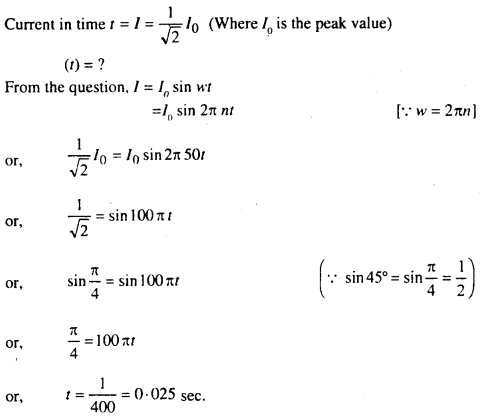Hence the required time = 0·025 sec.

Question 24.
The equation of an A.C. is I = 60 sin 500 πt. Calculate its frequency and r.m.s value of the current.
From question I = 60 sin 500 πt ………..(1)
But the equation of A.C. I = I0sin wt
= I0 sin 2π nt ………….(2)
Comparing eq. (1) and eq. (2),
we get I0 = 60 Amp.
and 2 ππ = 500 π
or, n = 250 Hz
Now, R.M.S. Value of the current = $$\frac{I_{0}}{\sqrt{2}}$$
$$\frac{60}{\sqrt{2}}$$ = 30$$\sqrt{2}$$
= 30 × 1·414 = 42·42 Amp.

Question 25.
The number of turns in the primary and secondary, coils of a transformer are 60 and 450 respectively. If the primary voltage is 10 v and the primary current is 2A, find the voltage and current in the secondary coil.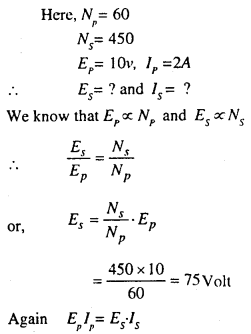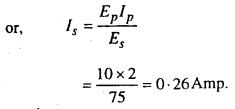Hence the voltage and the current in the secondary coil are 75 V and 0·26 Amp.

Question 26.
On an iron ring, there are 1000 tarns per metre of copper wire. When a current of 0.15 ampere flows through the wire, the magnetic field produced in the ring is 1·0 Tesla. Find the relative permeability and susceptibility of iron.
From question, No. of turns (n) = 1000
current (i) = 0·15 amp.
B = 1·0 Tesla
Relative permeability (μr) = ?
Magnetic susceptibility (k) = ?
Field produced due to current (H) = ni
= 100 × 0·15
= 150 A/mQuestion 27.
The maximum velocity with which an electron is emitted from a photocell is 3·66 × 106 m/s. Calculate the stopping potential. Mass and charge of electron are 9·1 × 10-31 kg and 1·6 × 10-19 coulomb respectively.
From question, u = 3·66 × 106 m/s
m = 9·1 × 10-31 kg
e = 1.6 × 10-19 coulomb
We know that kinective energy of electron = $$\frac{1}{2}$$mu2
Hence if V is the stopping potential then ev = $$\frac{1}{2}$$mu2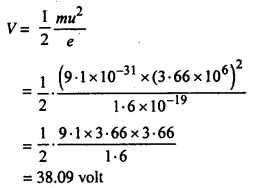Question 28.
Find the radio active constant or decay constant of an dement whose half life is 20 years.
From equation, T = 20 years
λ = ?
We know that
λ = $$\frac{0.6931}{T}$$
= $$\frac{0.6931}{20}$$
= 0.03465 per year

Question 29.
The half life period of radioactive substance is 30 days. Find how many time after only $$\frac{1}{4}$$ of the substance will remain, log4 e = 1.3863.
Let when t = 0, the number of atoms be N0.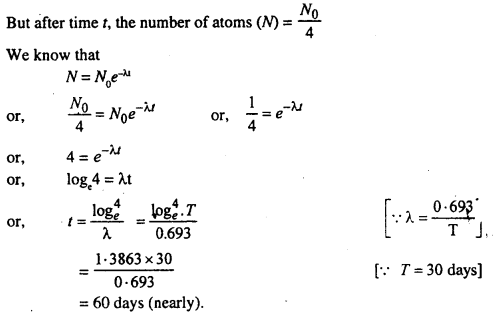Question 30.
Calculate the velocity of an electron if its energy is 728 eV. [1eV = 1.6 × 10-19 Joule and m = 9.1 × 10-31 kg.]
From question, Energy = 728 eV
= 728 × 1-6 × 10-19J
and m = 9·1 × 10-31 kg
We know that, Kinetic energy of electron = $$\frac{1}{2}$$mν2Question 31.
Calculate the velocity of electrons accelerated by a potential differeance of 1000 volts, charge of an electron = 1.6 × 10-19 and mass of an electron = 9 × 10-31 Kg.
Let be the velocity of the electron since the K.E. of the electron = work done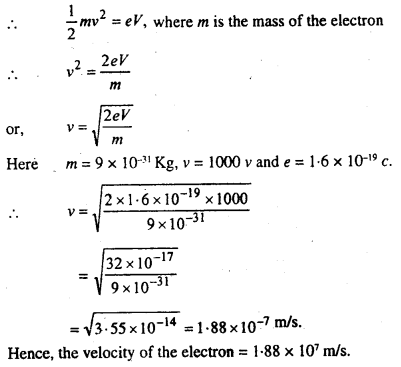Question 32.
The work function of sodium metal is 23 eV. What is the longest wave length of light that can cause photoelectron emission from sodium.
(h = 6.625 × 10-34J, c = 3 × 108 m/s. and 1eV = 1·6 × 10-19J
At threshold frequency the energy of the photon is equal to the work function.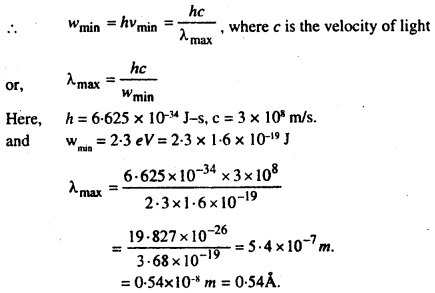Hence, the longest wavelength of light = 0.54Å.

Question 33.
An electron is placed in an electric field of intensity 2 × 104 volt/metre. What is the force acting upon the electron?
From question, Electric field of intensity = 2 × 104 v/m
Hence the force acting on unit charge in the field = 2 × 104 N/coulomb
∴ As the charge on the electron = 1.6 × 10-19 C
∴ Force acting on it = 1.6 × 10-19 × 2 × 104
= 3.2 × 10-15 Newton.

Question 34.
The torque required in a bar magnet for deflection of 30° from earth’s magnetic field is 5 AM2. Calculate the work done in deflecting the magnet again by 60° from earth’s field.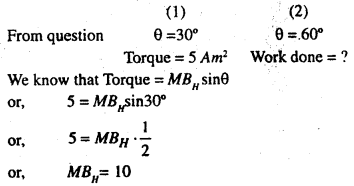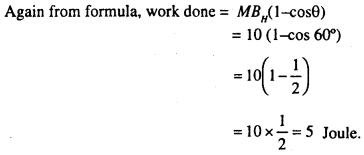Question 35.
At distance respectively 0.2 m and 0.4 m form the centre of a bar magnet on its axis, the ratio of the magnetic fields is $$\frac{25}{2}$$. Find the distance between the poles or length of the magnet
From question Fields d1 = 0.2 m, d2 = 0.4 m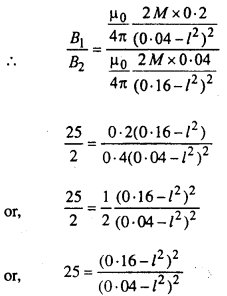or, 5 = $$\frac{0 \cdot 16-l^{2}}{0 \cdot 04-l^{2}}$$
or, 0.2 – 5l2 = 0.16 – l2
or, 0.2 – 0.16 = 5l2 – l2
or, 4l2 = 0 04
or, l2 = 0.01
or, l = 0.1m
∴ Distance between the poles = 2l
= 2 × 0.1
= 0.2 m

Question 36.
The apparent dip at a place 30° away from the magnetic meridian is 45°. Find the true dip.